# Binomial theorem Questions and Answers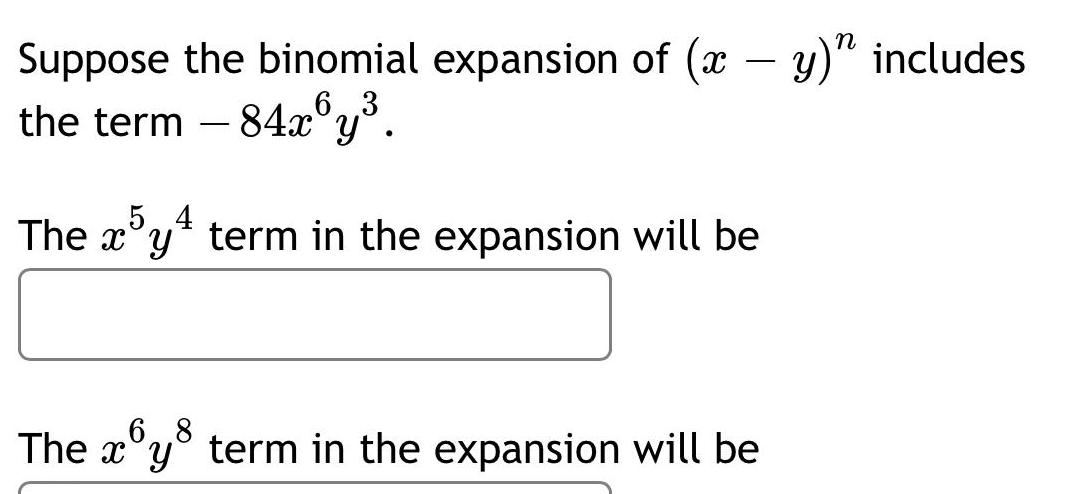Math
Binomial theorem
Suppose the binomial expansion of (x - y)^n includes the term - 84x^6y^3. The x^5y^4 term in the expansion will be The x^6y^8 term in the expansion will be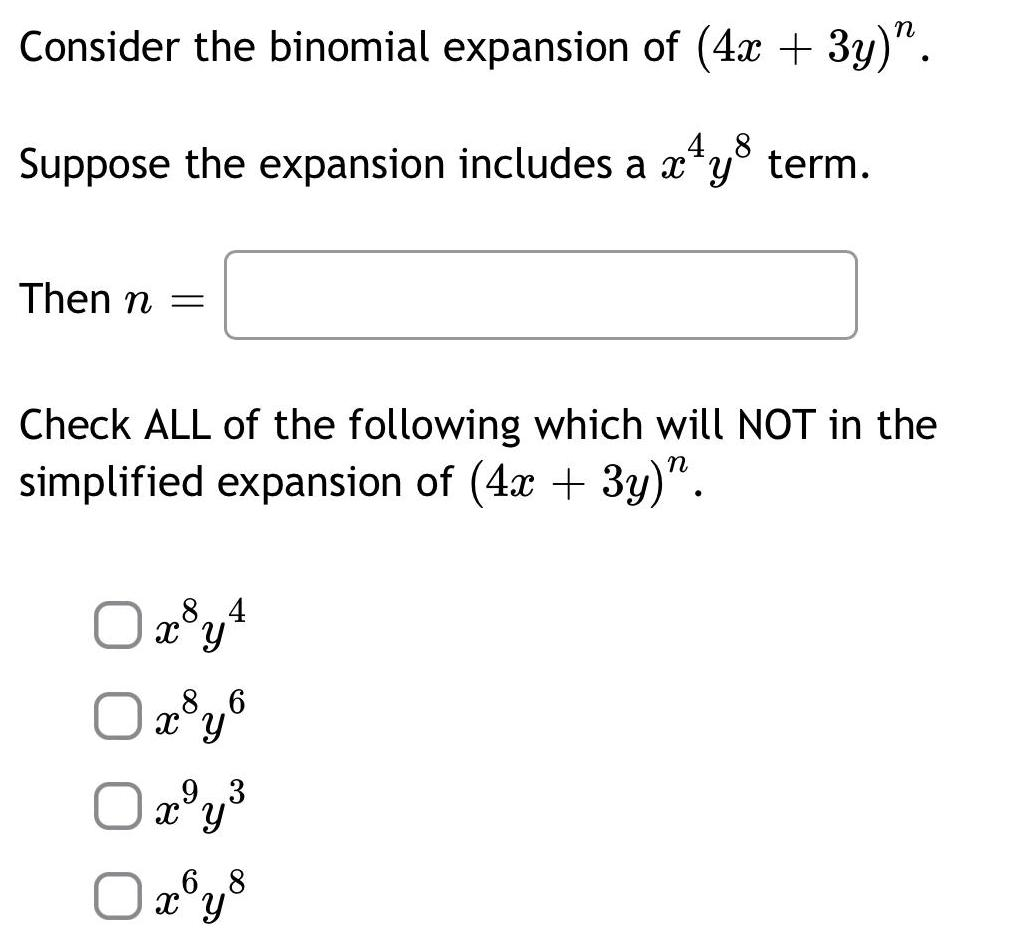Math
Binomial theorem
Consider the binomial expansion of (4x + 3y)^n. Suppose the expansion includes a x^4y^8 term. Then n = ___ Check ALL of the following which will NOT in the simplified expansion of (4x + 3y)^n. x^8y^4 x^8y^6 x^9y^3 x^6y^8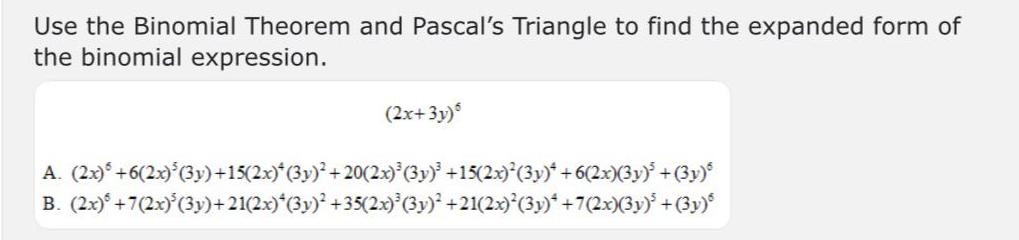Math
Binomial theorem
Use the Binomial Theorem and Pascal's Triangle to find the expanded form of the binomial expression. (2x+3y) A. (2x) +6(2x)³ (3y)+15(2x)*(3y)² +20(2x)³ (3y)³ +15(2x)² (3y)* +6(2x)(3y)³ +(3y) B. (2x)+7(2x)³ (3y)+21(2x)*(3y)² +35(2x)³ (3y)² +21(2x)²(3y)* +7(2x)(3y)³ +(3y)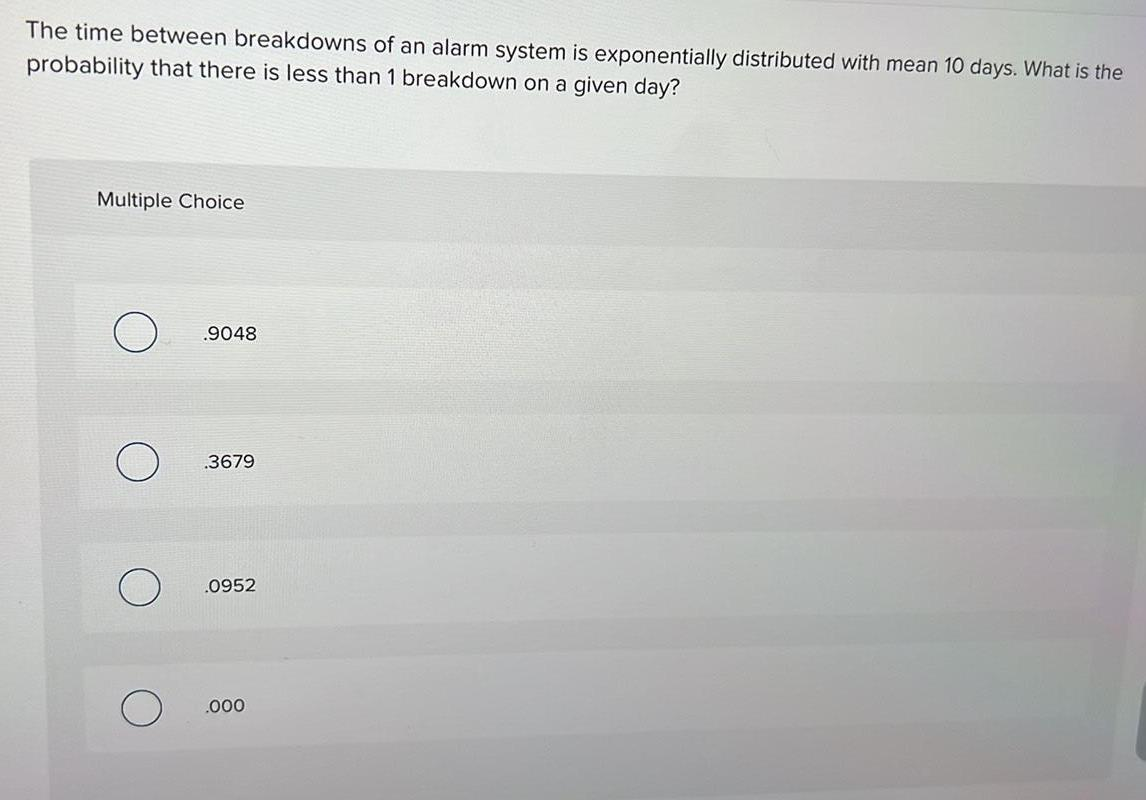Math
Binomial theorem
The time between breakdowns of an alarm system is exponentially distributed with mean 10 days. What is the probability that there is less than 1 breakdown on a given day? .9048 .3679 .0952 .000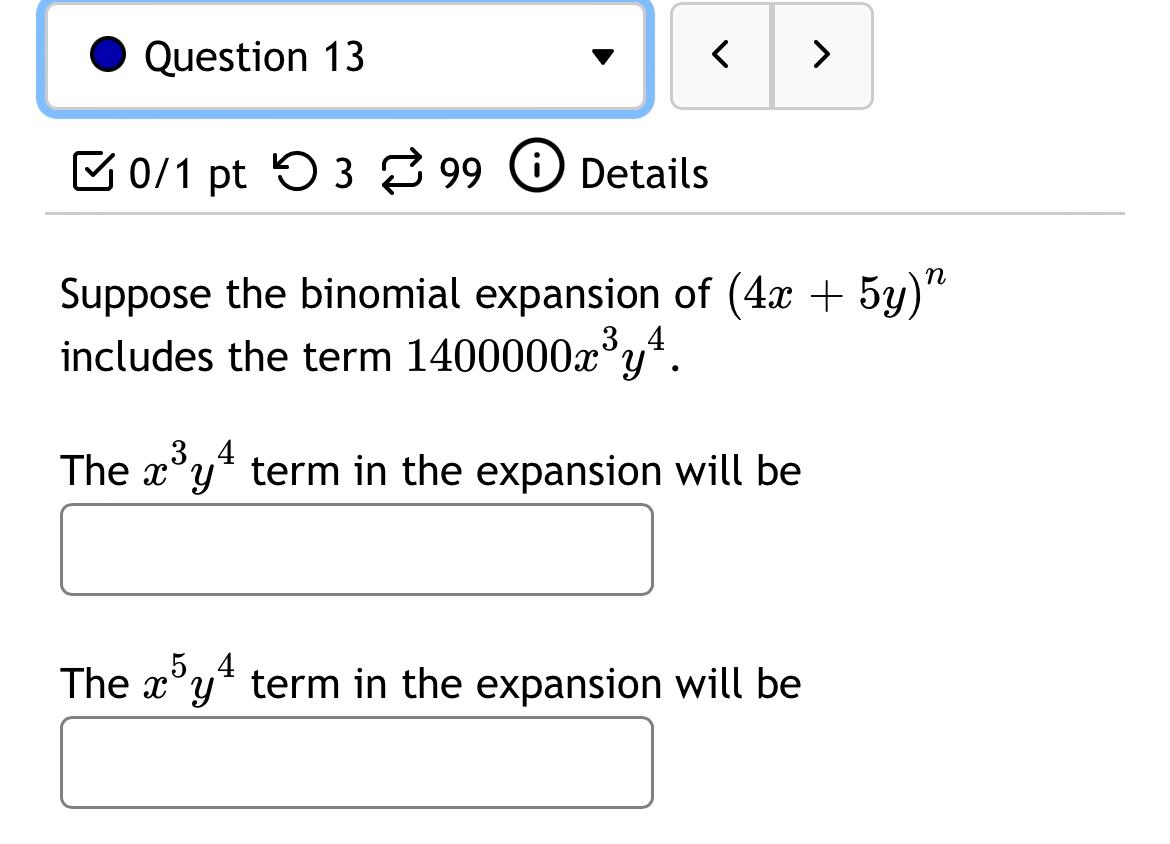Math
Binomial theorem
Suppose the binomial expansion of (4x + 5y)^n includes the term 1400000x³y⁴. (A) The x³y⁴ term in the expansion will be? (B) The x⁵y⁴ term in the expansion will be?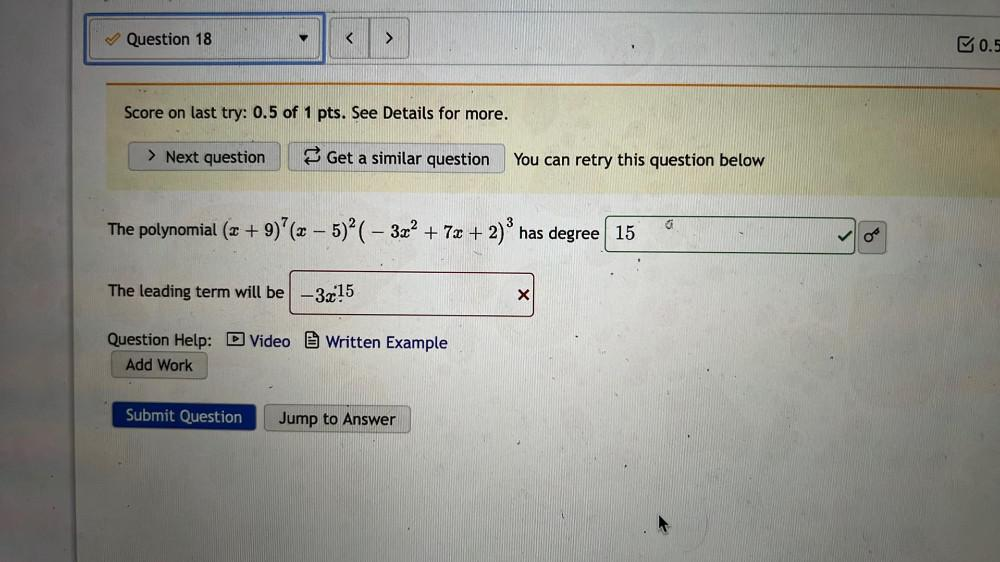Math
Binomial theorem
The polynomial (x + 9)⁷ (x – 5)² (- 3x² + 7x + 2)³ has degree? The leading term will be?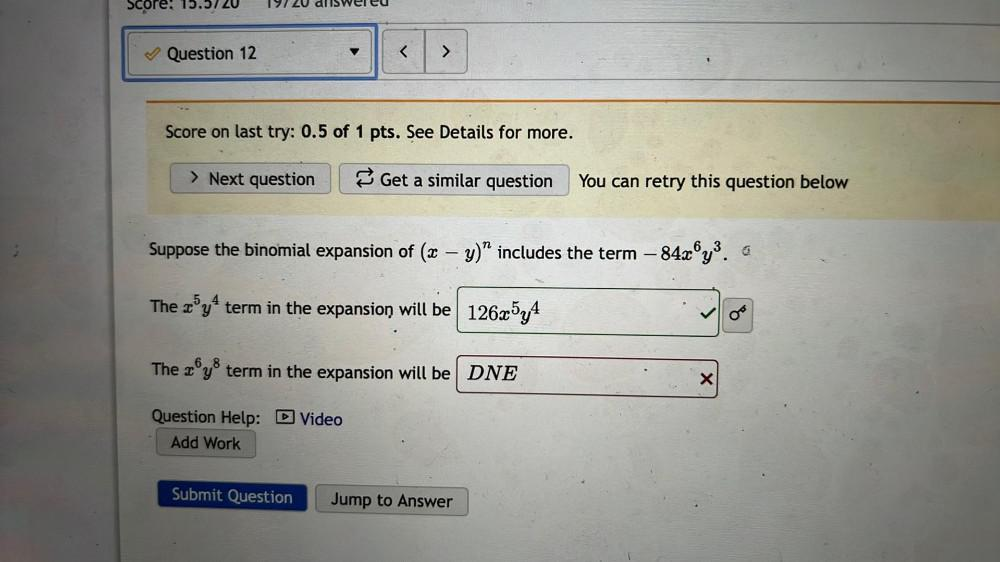Math
Binomial theorem
Suppose the binomial expansion of (x - y)n includes the term – 84x6y3 The x5y4 term in the expansion will be ___ The x6y8 term in the expansion will be ___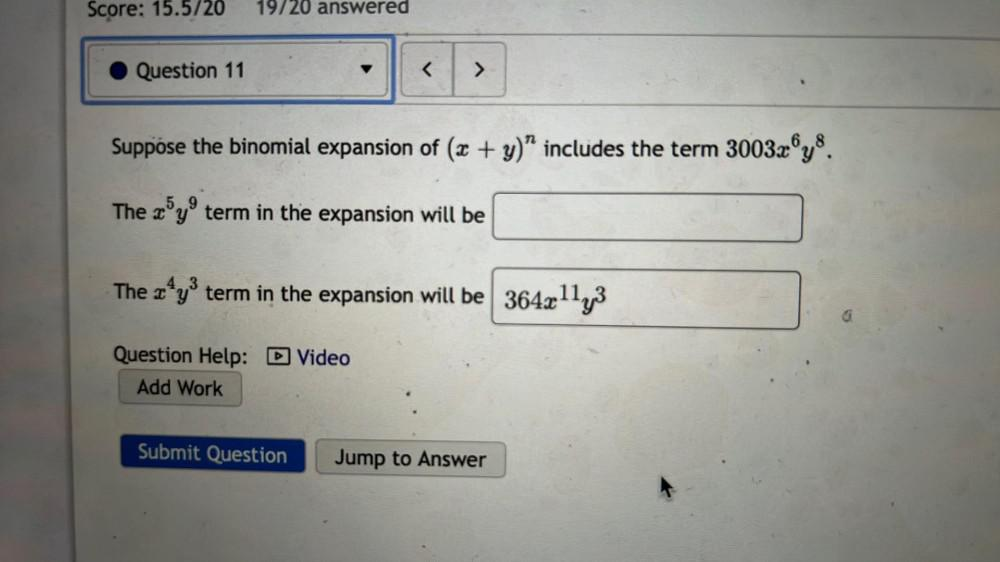Math
Binomial theorem
Suppose the binomial expansion of (x + y)^n includes the term 3003x⁶y⁸. The x⁵y⁹ term in the expansion will be? The x⁴y³ term in the expansion will be?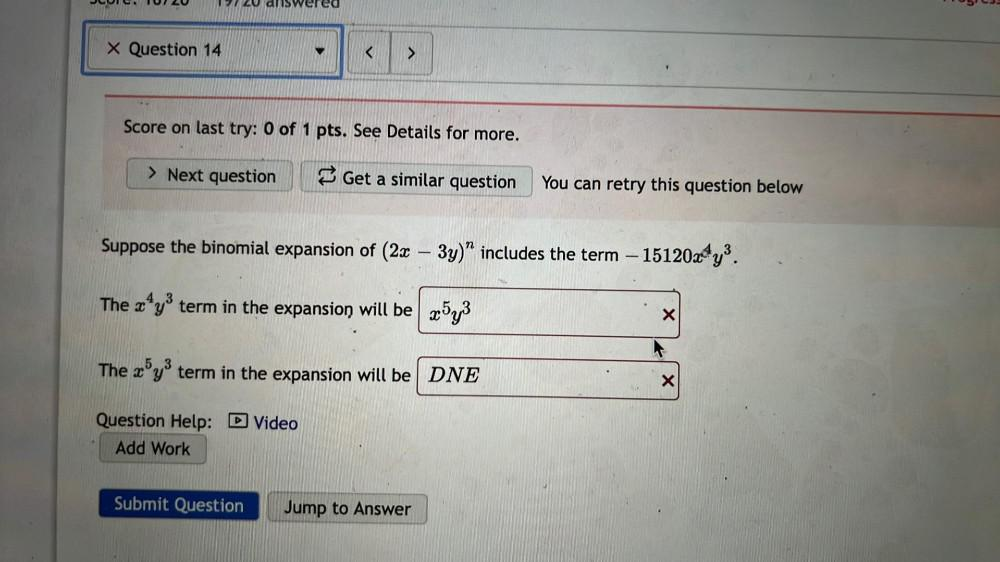Math
Binomial theorem
Suppose the binomial expansion of (2x – 3y)n includes the term –15120x4y3 The x4y3 term in the expansion will be The x5y3 term in the expansion will be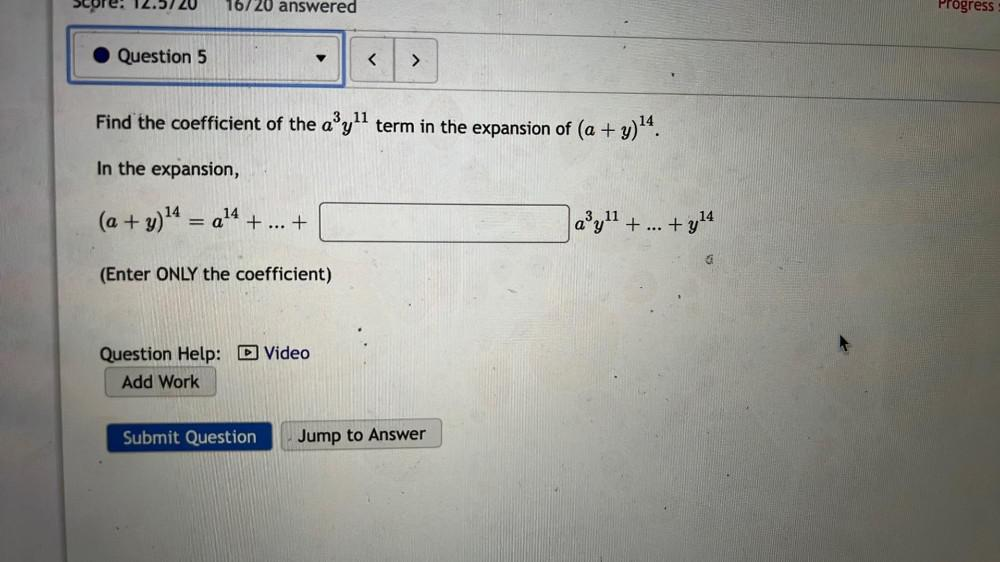Math
Binomial theorem
Find the coefficient of the a³y¹¹ term in the expansion of (a + y)¹⁴. In the expansion, (a + y)¹⁴ = a¹⁴ + ... +___________ a³y¹¹ + ... +y¹⁴ (Enter ONLY the coefficient)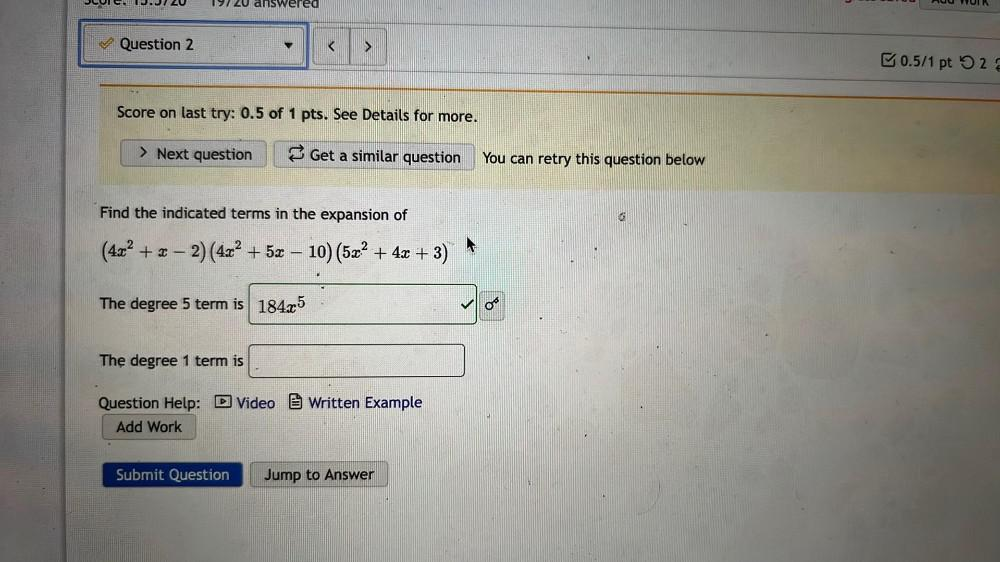Math
Binomial theorem
Find the indicated terms in the expansion of (4x² + x - 2)(4x² + 5x – 10) (5x² + 4x + 3) The degree 5 term is ___ The degree 1 term is ___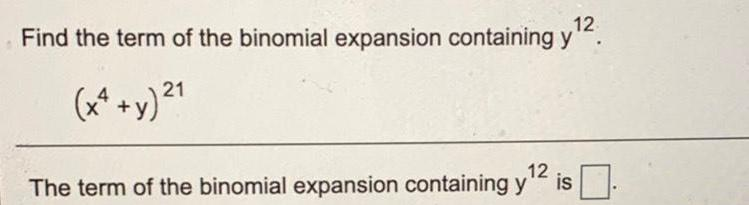Math
Binomial theorem
Find the term of the binomial expansion containing y^12. (x^4+y)^21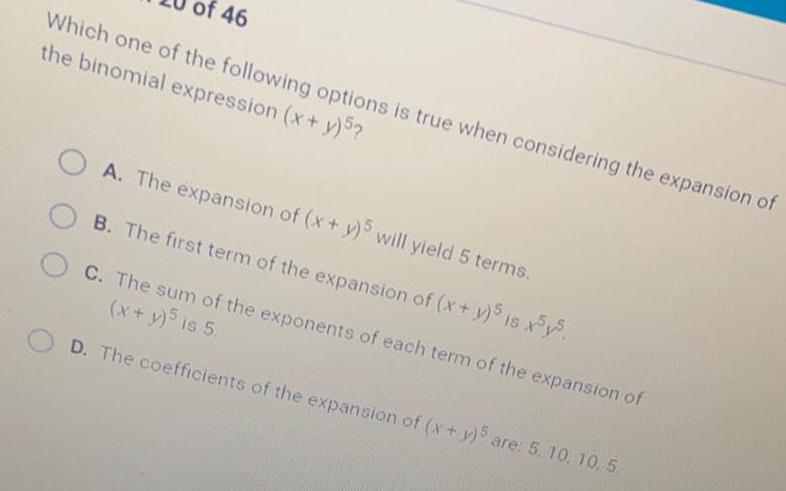Math
Binomial theorem
Which one of the following options is true when considering the expansion of the binomial expression (x + y)5? A. The expansion of (x + y)5 will yield 5 terms. B. The first term of the expansion of (x + y)5 is x5y5. C. The sum of the exponents of each term of the expansion of (x + y)5 is 5. D. The coefficients of the expansion of (x + y)5 are: 5, 10, 10, 5.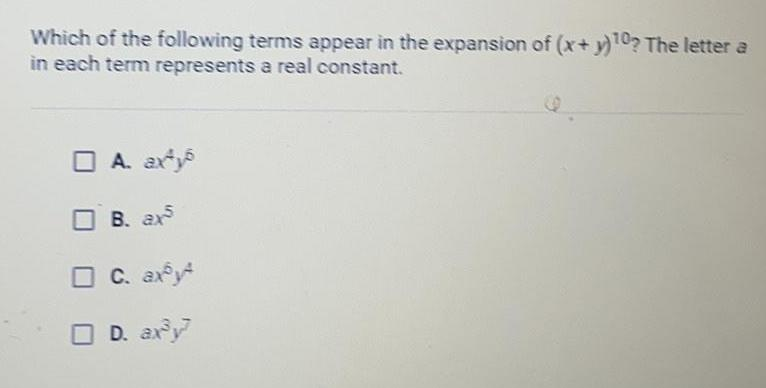Math
Binomial theorem
Which of the following terms appear in the expansion of (x+y)^10? The letter a in each term represents a real constant. A. ax^4y^6 B. ax^5 C. ax^6y^4 D. ax³y^7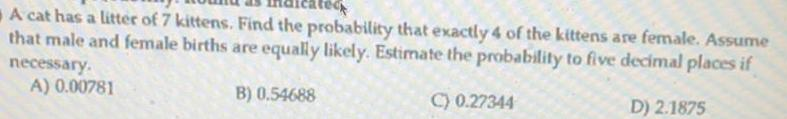Math
Binomial theorem
A cat has a litter of 7 kittens. Find the probability that exactly 4 of the kittens are female. Assume that male and female births are equally likely. Estimate the probability to five decimal places if necessary. A) 0.00781 B) 0.54688 C) 0.27344 D) 2.1875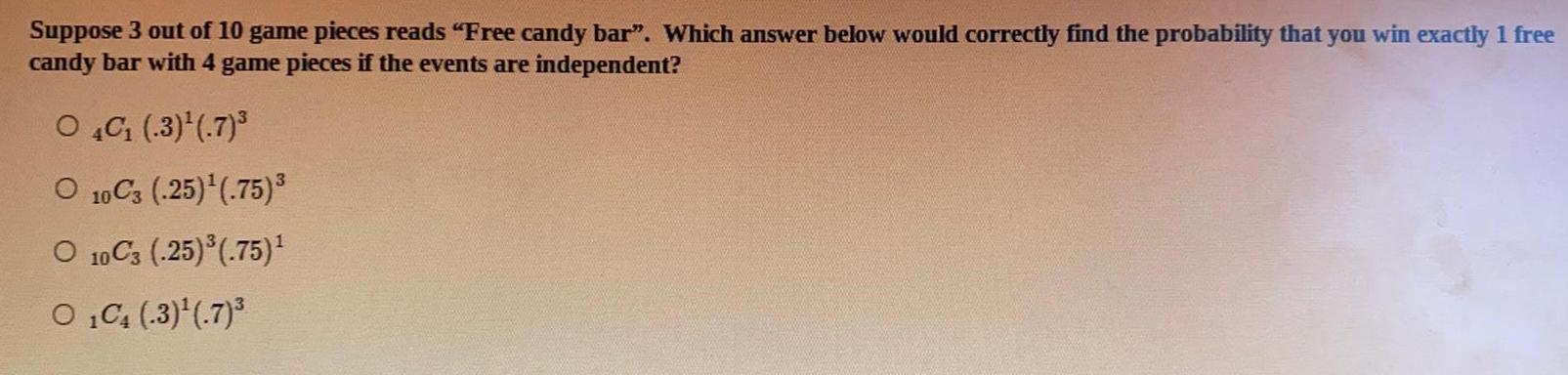Math
Binomial theorem
Suppose 3 out of 10 game pieces reads "Free candy bar". Which answer below would correctly find the probability that you win exactly 1 free candy bar with 4 game pieces if the events are independent? 4C₁ (.3)¹ (7)³ 10C3 (-25)¹(.75)³ 10C3 (.25) ³ (.75)¹ 1C₁ (-3)¹ (7)³